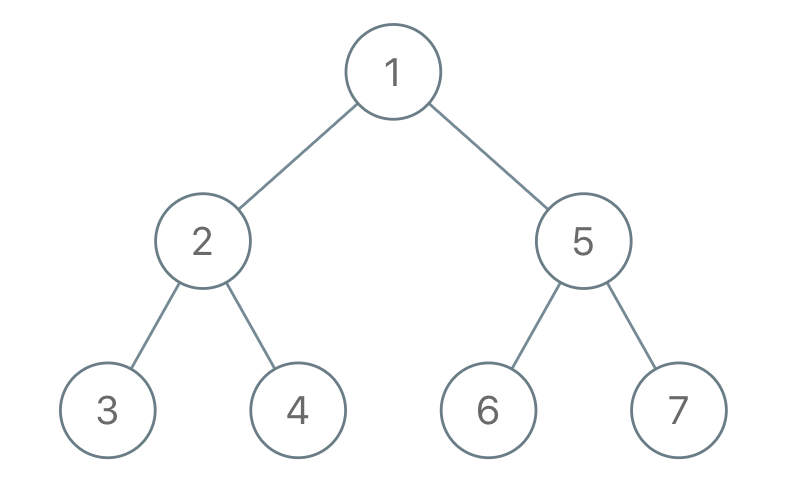Problem list:

## 1025. Divisor Game

### Problem

Alice and Bob take turns playing a game, with Alice starting first.

Initially, there is a number `N` on the chalkboard. On each player's turn, that player makes a move consisting of:

• Choosing any `x` with `0 < x < N` and `N % x == 0`.
• Replacing the number `N` on the chalkboard with `N - x`.

Also, if a player cannot make a move, they lose the game.

Return `True` if and only if Alice wins the game, assuming both players play optimally.

Example 1:

Example 2:

Note:

1. `1 <= N <= 1000`

https://leetcode.com/contest/weekly-contest-132/problems/divisor-game/

### Solution

The first though was "dynamic programming"...

Is this an easy one? Yes… Then I found that we just need to check if the given number is even or not.

Proof:

Firstly, we can prove that the one who gets input `3` will lose the game.

Consider if `N` is odd, Alice can only have an odd `x`, which makes `N-x` even for Bob. Bob choose whatever an odd number to give another odd number to Alice. Keep going like this, Alice will finally be given a `3`, she then loses the game.

Consider if `N` is even, she can play this trick to win the game.

## 1026. Maximum Difference Between Node and Ancestor

### Problem

Given the `root` of a binary tree, find the maximum value `V` for which there exists different nodes `A` and `B` where `V = |A.val - B.val|` and `A` is an ancestor of `B`.

(A node A is an ancestor of B if either: any child of A is equal to B, or any child of A is an ancestor of B.)

Example 1:

Note:

1. The number of nodes in the tree is between `2` and `5000`.
2. Each node will have value between `0` and `100000`.

https://leetcode.com/contest/weekly-contest-132/problems/maximum-difference-between-node-and-ancestor/

### Solution

My first try used two nested recursions.

Actually one depth-first search is enough (by keeping the max and min value):

## 1027. Longest Arithmetic Sequence

### Problem

Given an array `A` of integers, return the length of the longest arithmetic subsequence in `A`.

Recall that a subsequence of `A` is a list `A[i_1], A[i_2], ..., A[i_k]` with `0 <= i_1 < i_2 < ... < i_k <= A.length - 1`, and that a sequence `B` is arithmetic if `B[i+1] - B[i]` are all the same value (for `0 <= i < B.length - 1`).

Example 1:

Example 2:

Example 3:

Note:

1. `2 <= A.length <= 2000`
2. `0 <= A[i] <= 10000`

https://leetcode.com/contest/weekly-contest-132/problems/longest-arithmetic-sequence/

### Solution

The idea is to keep track of two numbers as the first two elements in the sequence using two nested for loop, and then use another for loop interating from the next number to check how many numbers match this sequence:

## 1028. Recover a Tree From Preorder Traversal

### Problem

We run a preorder depth first search on the `root` of a binary tree.

At each node in this traversal, we output `D` dashes (where `D` is the depth of this node), then we output the value of this node. (If the depth of a node is D, the depth of its immediate child is D+1. The depth of the root node is 0.)

If a node has only one child, that child is guaranteed to be the left child.

Given the output `S` of this traversal, recover the tree and return its `root`.

Example 1:Example 2:Example 3:

• The number of nodes in the original tree is between `1` and `1000`.
• Each node will have a value between `1` and `10^9`.